# Put-Call Parity

Demonstrates the Relationship Between European Put and Call Options

## What is the Put-Call Parity?

The put-call parity is an important concept in options pricing which shows how the prices of puts, calls, and the future underlying asset must be consistent with one another. This equation establishes a relationship between the price of a European call and put option which have the same underlying asset. For this relationship to work, the call and put option must have an identical expiration date and strike price. The put-call parity relationship shows that a portfolio consisting of a long call option and a short put option should be equal to a forward contract with the same underlying asset, expiration, and price as the strike. This equation can be rearranged to show alternative ways of viewing this relationship. For example, another way this relationship can be interpreted would be that a portfolio of a short call option and a long put option should be equal to a long position on the strike price and short position of the underlying asset.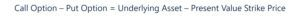### Quick Summary of Points

• The put-call parity is an important relationship between the prices of puts, calls, and the underlying asset
• This relationship is only true for European options with identical strike prices, maturity dates, and underlying assets
• The equation states that a long position on the call and a short position on the put should equal a long position on the underlying asset and a short position on the strike price
• The put-call parity can be used to identify arbitrage opportunities in the market

### Put-Call Parity Excel Calculator

Below, we will go through an example question involving the put-call parity relationship. This can easily be done on excel. To download the put-call parity calculator, check out CFI’s free resource: Put-Call Parity Calculator

### Interpreting the Put-Call Parity

To better understand the put-call parity, let us consider a hypothetical situation where you buy a call option for \$10 with a strike price of \$100 and maturity date of one year, as well as sell a put option for \$10 with an identical strike price and expiration. According to the put-call parity, that would be equivalent to buying the underlying asset and borrowing an amount equal to the strike price discounted to today. The spot price of the asset is \$100 and we make the assumption that at the end of the year the price is \$110, so does the put-call parity hold?

If the price goes up to \$110, you would exercise the call option. You paid \$10 for it but you can buy the asset at the strike price of \$100 and sell it for \$110, so you net \$0. You have also sold the put option. Since the asset has increased in market value, the put option will not be exercised by the buyer and you pocket the \$10. That leaves you with \$10 from this portfolio.

What is the portfolio consisting of the underlying asset and short position on the strike price worth at the expiration date? Well, if you had invested in the asset at the spot price of \$100 and it ended at \$110, and you had to pay back the strike price at maturity from the amount you borrowed which would be \$100, the net amount would be \$10. We see that these two portfolios both net to positive \$10 and the put-call parity holds.

### Why is the Put-Call Parity Important?

The put-call parity is important to understand because this relationship must hold in theory. With European put and calls, if this relationship does not hold then that leaves an opportunity for arbitrage. Rearranging this formula, we can solve for any of the components of the equation. This allows us to create a synthetic call or put option. If a portfolio of the synthetic option costs less than the actual option, based on put-call parity, a trader could employ an arbitrage strategy to profit.

### What is the Put-Call Parity Equation?

As mentioned above, the put-call parity equation can be written a number of different ways and rearranged to make varying inferences. A couple of common ways it is expressed is as follows: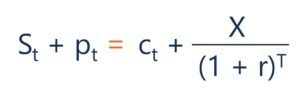St + pt = ct + X/(1 + r)^T

The above equation shown in this combination can be interpreted as a portfolio holding a long position in both the underlying asset and a put option should equal a portfolio holding a long position in both the call option and the strike price. According to the put-call parity this relationship should hold or else an opportunity for arbitrage would exist.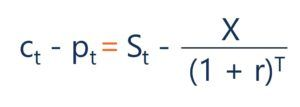ct – pt = St – X/(1 + r)^T

In this version of the put-call parity, a portfolio that holds a long position in the call, and a short position in the put should equal a portfolio consisting of a long position in the underlying asset and a short position of the strike price.

For the above equations, the variables can be interpreted as:

• St = Spot Price of the Underlying Asset
• pt = Put Option Price
• ct = Call Option Price
• X/(1 + r)^T =  Present Value of the Strike Price, discounted from the date of expiration
• r = The Discount Rate, often the Risk-Free Rate

The equation can also be rearranged and solved for a specific component. For example, based on the put-call parity, a synthetic call option can be created. The following shows a synthetic call option: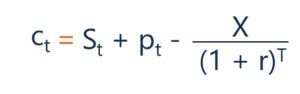ct = St + pt – X/(1 + r)^T

Here we can see that the call option should be equal to a portfolio with a long position on the underlying asset, a long position on the put option and a short position on the strike price. This portfolio can be thought of as a synthetic call option. If this relationship doesn’t hold then arbitrage exists. If the synthetic call was less than the call option, then you could buy the synthetic call and sell the actual call option to profit.

### Put-Call Parity – European Call Option Worked Example

Let us now consider a question involving the put-call parity. Suppose a European call option on a barrel of crude oil with a strike price of \$50 and a maturity of one-month, trades for \$5. What is the price of the put premium with identical strike price and time until expiration, if the one-month risk-free rate is 2% and the spot price of the underlying asset is \$52?

Here we can see the calculation that would be used to find the put premium: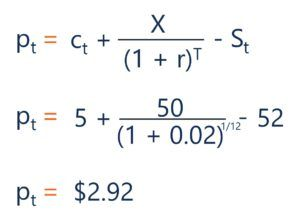These calculations can also be done in Excel. The following shows the solution to the above question done in excel: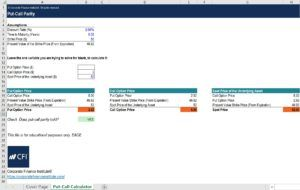Thank you for reading CFI’s article on the put-call parity. If you would like to learn more about related concepts check out CFI’s other resources:

• Options: Calls and Puts
• Option Pricing Models
• Arbitrage
• Derivatives

### Financial Analyst Training

Get world-class financial training with CFI’s online certified financial analyst training program!

Gain the confidence you need to move up the ladder in a high powered corporate finance career path.

Learn financial modeling and valuation in Excel the easy way, with step-by-step training.